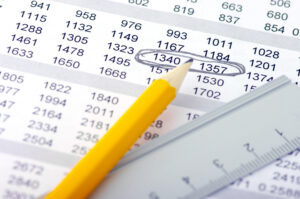##How to work with dates in Excel

Before you can calculate age, you have to know how to work with dates. Excel allows you to format dates in a number of ways. Excel also contains a number of different date and time functions to help you work with and manipulate date and time functions. To work effectively with date and time functions, you need to have a basic understanding of how Excel stores dates and times. For a basic excel course, excel for beginners will teach you the basics of working with Excel and it will give you great tips and ideas to make your spreadsheet life a lot easier.

## How to format dates in Excel

To create a date field in Excel and display the date correctly, you need to choose the appropriate date format for the cell. To format a cell as a date, enter the date using “/” character between the day month and year. For example, the 3rd of March 2013 would be entered into the cell as 03/03/2013. Excel generally assumes that format indicates a date, but if for some reason your cell format is specified as something else, then it is simple to change your call value into a date using the date format available for the cell. Excel stores dates as numbers so the format you choose to display your date will not affect date calculations that you make based on that date.

## Practical Example to work out Age in Excel in Years

To show you how to work with dates in Excel, we have prepared a practical step-by-step example of how to work out age in Excel. Our example will be based on the following sample data:To work out age, you first need to decide on the criteria for the calculation. In our first example, we will work out how old everyone was at the family reunion that we all attended on the 30th of November 2013. So we enter the “end” date for the calculation into our spreadsheet, under the calculation date column:To show you that the format of the date does not affect the calculation, we formatted the calculation date using the “long date” format and the birth date using the “short date” format.

Now that we have the two dates entered, we can use various formulas to work out age in years.

## How to use mathematical formulas to work out age in Excel

Excel stores dates as a number. You can therefore work out the age in years, months or even days using a mathematical formula. To use the above data to calculate the age each family member in days you can subtract the calculation date from the birth date and Excel will work out the number of days between each date.

If you want the age in years, you need to divide the days by 365.25 since there are 365 days per year and a leap year every four years. To use the formula, type the following into the Age cell and then copy the formula for each record.The resulting spreadsheet will look like this:Excel 2013 made easy is designed to create a strong foundation for using Excel in business. Sign up for this course today to learn to harness the power of Excel.

## How to use the today function to work out age

If you wanted an updated status of each person’s age on a daily basis then you could use the TODAY() function to keep the calculation date up to date with today’s date. To access the TODAY() function, you can either type the function into the cell manually or you can insert the function via the Formula tab by selecting TODAY() from the Date & Time functions. To type it directly within the cell, type =TODAY() and then press enter. The result will look like this:## Calculating age using the YEAR function in Excel

The YEAR() function returns the Year portion of a date within Excel. Now that we have two dates we can use the YEAR() function to calculate the difference in years between the two dates. The formula will look like this:You can either type the function into the cell directly or use the Formula tab to add the formula. The Formula tab opens the function argument window which will help you enter the formula. To add the Year function via the Formula tab, click the Formula tab, then select the YEAR function from the Date & Time functions. The following argument window will open:The result of the above formula will be as follows:## How to use the DATEDIF function to calculate age in Excel

The DATEDIF function in excel returns the number of years, months or days between two dates. The DATEDIF function is a little more accurate than using a mathematical formula to calculate age since DATEDIF takes things like leap years into account.

The syntax of the DATEDIF function is:

=DATEDIF( StartDate ; EndDate; “Time”) where time can be set as “Y” for years, “YM” for months or “MD” for days.

The formula for our worksheet will look as follows:And the results will look like this:You can also add “years” to the DATEDIF function to format the result even further. Here is the formula to add the statement to the result:And this is the resulting worksheet:## Conclusion

Excel is the world’s top spreadsheet application and one of the reasons for its popularity is the fact that Excel offers its users immense power to manipulate data using the built-in functions and VBA code. There is often more than one way to achieve the same results in Excel and understanding which Excel functions are available, and how they work, will unlock the power of Excel for you. For an advanced course in Excel, why not sign up for Rainman Excel Essentials today and learn Excel by following their fun Excel tutorials online?

Page Last Updated: February 2014